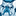# QlikView App Dev

Discussion Board for collaboration related to QlikView App Development.

Announcements
Now Live: Qlik Sense SaaS Simplified Authoring – Analytics Creation for Everyone: READ DETAILS
cancel
Showing results for
Did you mean:Contributor II

## Greater than or equal in set analysis with variable

Hi,

I'm stuck on a function and I haven't found a solution on a community yet. I suppose I'm not the first one to have this kind of issue so my apologies if this has been posted before.

The following function works as expected:

=Count(DISTINCT {\$<\$(var1)={"=Sum({\$<MonthSerial={\$(vMin)}>}\$(Sales))<=5000"}>*<\$(var1)={"=Count(DISTINCT {\$<MonthSerial={\$(vMin)}>}[Hierarchy Lev1])<=4"}>}\$(var1))

My variable vMin contains a single integer. I would like to add the condition the MonthSerial is >= vMin and not only =. This is what I wrote.

=Count(DISTINCT {\$<\$(var1)={"=Sum({\$<MonthSerial={">=\$(vMin)"}>}\$(Sales))<=5000"}>*<\$(var1)={"=Count(DISTINCT {\$<MonthSerial={">=\$(vMin)"}>}[Hierarchy Lev1])<=4"}>}\$(var1))

The result is null all the the time and I do not see what is wrong in the syntax. Can somebody help me with this?

1 Solution

Accepted SolutionsMVP

Hi @Jjotavve, that's because the double quotes of monthseriel is closing the previous double quotes, not opening a new one, try with different symbols like an accent, o double-double quotes:

=Count(DISTINCT {\$<\$(var1)={"=Sum({\$<MonthSerial={´>=\$(vMin)´}>}\$(Sales))<=5000"}>*<\$(var1)={"=Count(DISTINCT {\$<MonthSerial={"">=\$(vMin)""}>}[Hierarchy Lev1])<=4"}>}\$(var1))

2 RepliesMVP

Hi @Jjotavve, that's because the double quotes of monthseriel is closing the previous double quotes, not opening a new one, try with different symbols like an accent, o double-double quotes:

=Count(DISTINCT {\$<\$(var1)={"=Sum({\$<MonthSerial={´>=\$(vMin)´}>}\$(Sales))<=5000"}>*<\$(var1)={"=Count(DISTINCT {\$<MonthSerial={"">=\$(vMin)""}>}[Hierarchy Lev1])<=4"}>}\$(var1))Contributor II
Author

Hi @rubenmarin, I figured it would come from this and I tried putting triple-double quotes, but not double-double quotes. Ah, I was so close, but your solution works, thanks!